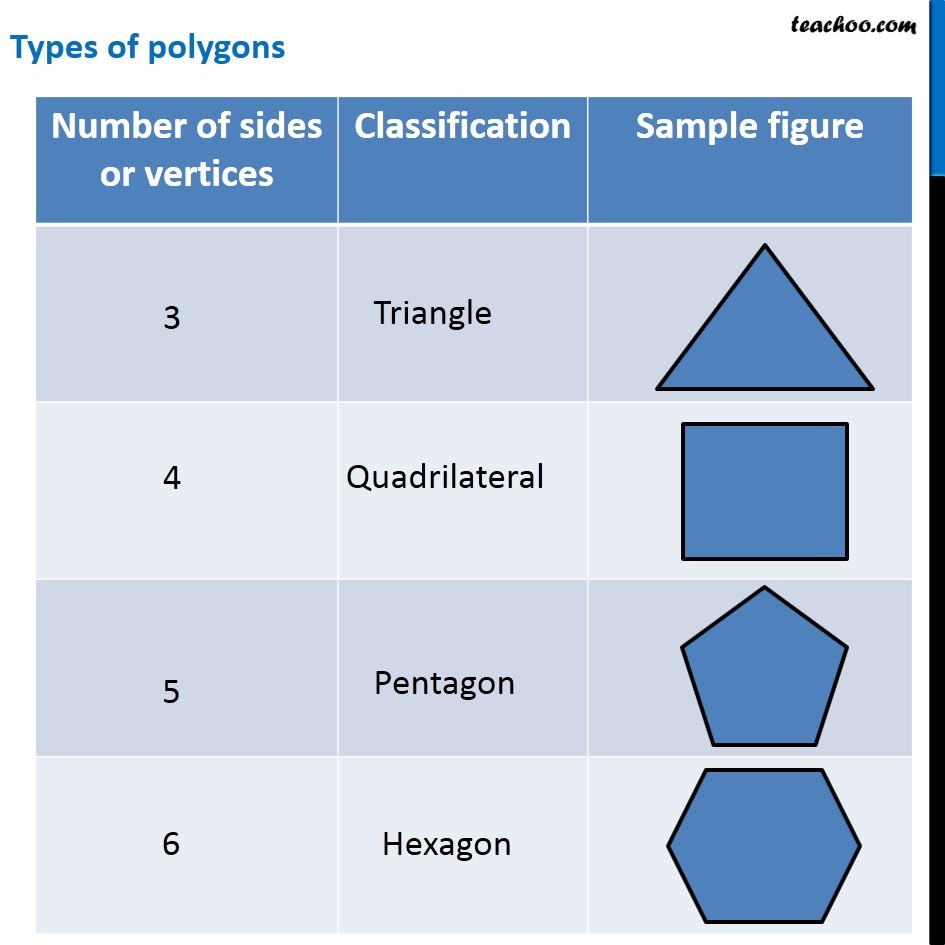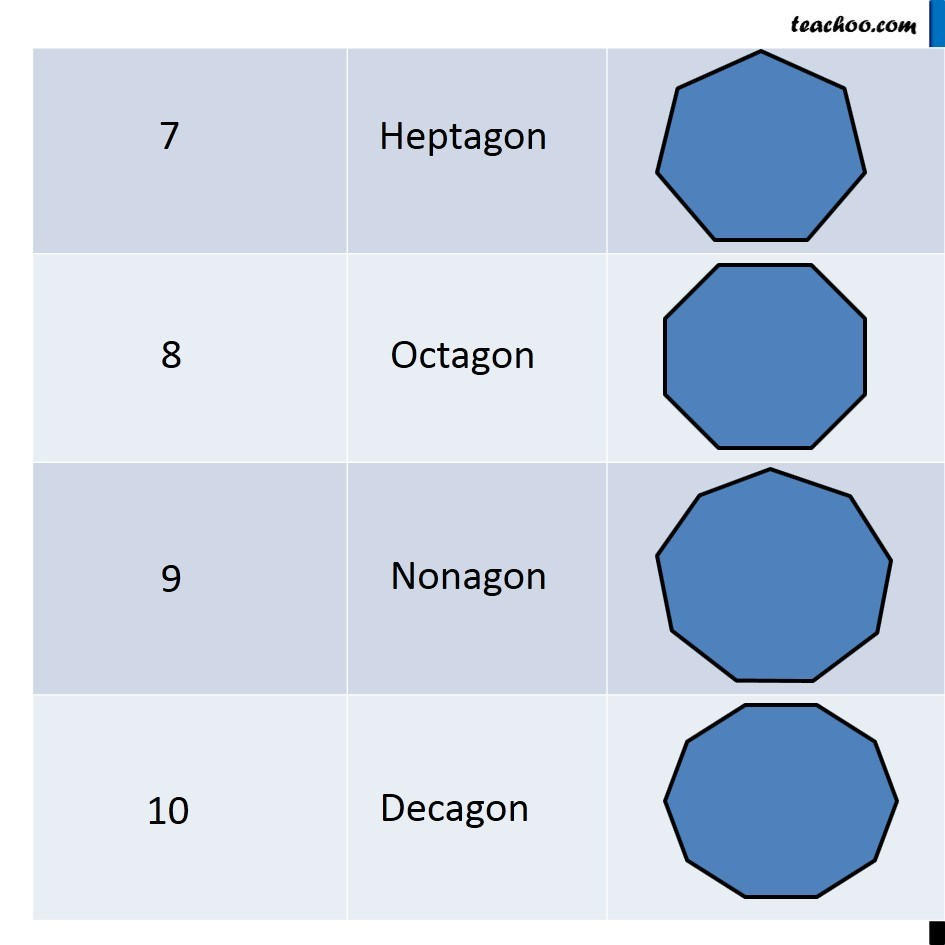Polygons

Chapter 5 Class 6 Understanding Elementary Shapes
Concept wise

Here, we see different types of polygons and their images

Note : Minimum number of vertices of a polygon is 3, anything less than 3 is not a polygonExamples of polygons are

• Triangle has 3 vertices
• Pentagon has 5 vertices
• Hexagon has 6 vertices
• Heptagon has 7 vertices
• Octagon has 8 vertices
• Nonagon has 9 vertices
• Decagon has 10 vertices

Learn in your speed, with individual attention - Teachoo Maths 1-on-1 Class